# Measurement II

### Guest Account

Attempt Form Two Physics Questions
Guest Account

# Measurement II

#### Measuring length using vernier callipers.

- Vernier callipers is used when higher accuracy in measurement is required and this cannot be done using a metre rule.
- Vernier callipers has two scales; main scale and vernier scale. Outside jaws are used to measure both lengths and external diameters, inside jaws for measuring internal diameters while the tail is used for measuring depths of cavities.
- main scale is divided into cm and mm.
- The vernier scale is divided into 10 equal divisions of 0.9 mm each. The accuracy of vernier callipers is 0.10 mm.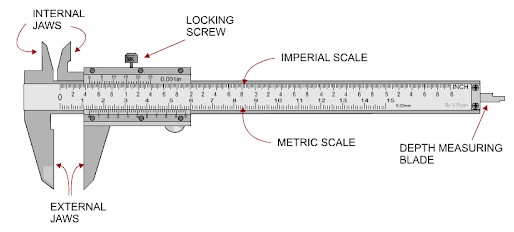The reading is taken in two steps:
1. The main scale is read at zero mark of the vernier scale. The values given in cm.
2. The vernier is read at the position where a mark on the vernier scale is exactly lined up with a mark on the main scale. The values are given as a two decimal of a cm.
Example 1
1. Give the reading in the following diagram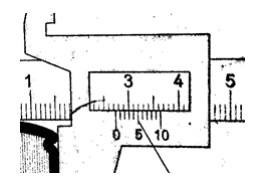Solution
Main scale reading: - 2.7 cm
Vernier scale reading: - 0.04 cm
Adding both we get 2.74 cm

Example 2
2. What is the reading of the vernier callipers shown below?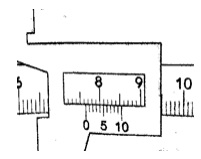Solution
Main scale reading - 7.6 cm
Vernier scale reading - 0.04 cm

#### Micrometer screw gauge

- It is a device used to measure small lengths. It has an accuracy of 0.01 mm.
- It has two scales; the sleeve scale and thimble scale.
- The sleeve scale is divided into upper and lower scales with the upper division in mm and lower divisions in 0.5 mm.
- Thimble scale is divided into 50 equal divisions each division consisting of 0.01 mm.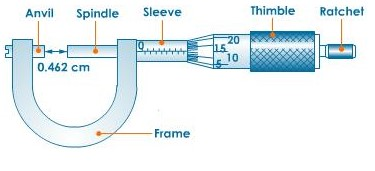The reading is taken in two steps:
a) The reading on the sleeve scale is read ta the point where it touches the edge of the thimble in mm and half mm.
b) The thimble scale is read at the point where the centre line of the sleeve is parallel to the thimble scale division.
Example 2
1. Give the reading in the following.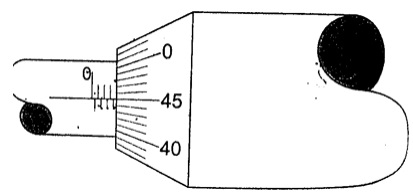Solution
Adding up we get 3.95 mm.
Example 2
2. What is the reading in the following micrometer screw gauge?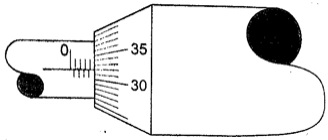Solution
Sleeve scale reading – 4.0 mm.
Thimble scale reading - 0. 32 mm.
Adding up the two we get 4.32 mm.

## Calculating the size of a molecule

-Both the volume and area of a drop can be calculated using the following formulas;
Volume = 4/3 Πr3
and
Area = Πr2h.
Example 1
1. A drop of olive oil, whose volume is 0.12 mm3, was placed on a surface of clean water. The oil spread and formed a patch of area 6.0 x 104 mm2. Estimate the size of the olive oil.
Solution
Volume = 0.12 mm3
Area of the oil patch = 6.0 x 104 mm2
Volume = area x thickness of the patch, therefore Thickness of the oil patch = volume / area.
= 0.12 ÷ 6.0 x 104= 2.0 x 10-6 mm or 2.0 x 10-9 m.

Example 2
2. Suppose an oil drop has a volume of 0.10 mm3 and forms a film with a radius of 10 cm. Calculate, the thickness of the oil film.
Solution
Area of the film = Πr2
= 3.14 x 10 x 10 = 314 cm2= 31,400 mm2.
Thickness of the oil film = volume ÷ area, hence 0.10 / 31,400 = 3.0 x 10-6 mm.

(The thickness of the oil film is called upper limit to the size of molecule because the molecule cannot be bigger than the thickness of the oil film)